# Hydrodynamic investigation of slurry flows in horizontal narrow rectangular channels

Hydrodynamic investigation of slurry flows in horizontal narrow rectangular channels

Libo Ren Xinqiang Zhao Shaofeng Zhang*

School of Chemical Engineering, Hebei University of Technology, Tianjin 300132, China

Shanghai Heat Transfer Equipment CO., LTD, Shanghai 201508, China

Corresponding Author Email:
1986037@hebut.edu.cn
Page:
730-736
|
DOI:
https://doi.org/10.18280/ijht.350406
|
Accepted:
|
Published:
31 December 2019
| Citation

OPEN ACCESS

Abstract:

Despite the extensive research on the liquid-solid two-phase flow in horizontal circular tubes, there is rarely any discussion over the liquid-solid two-phase hydrodynamics and the solid phase diffusion features in horizontal narrow rectangular channels. To make up for the gap, this paper employs the experimental method and CFD-DEM to investigate the hydrodynamics of glass beads-water slurry flows in a 12-mm tall horizontal rectangular channel, and explore the slurry flow features of the system at the velocity of 1.0m/s. The main conclusions are as follows. First, a dilute disperse phase and a dense cluster phase were formed; Second, in the vertical direction, the solid concentration is positively correlated with the degree of asymmetry of solid velocity profiles, but negatively with that of solid concentration profiles; Third, three distinct regions in the slurry flows exhibited different standard variances of solid velocity and solid concentration. This research provides new insights into the liquid-solid two-phase flow in horizontal narrow rectangular channels.

Keywords:

CFD-DEM, experiment, slurry, horizontal narrow rectangular channel

1. Introduction

The liquid-solid flow, also known as slurry flow, is commonplace in industrial applications. In the past years, considerable efforts have been devoted to several important indicators of slurry flow in horizontal pipe, such as pressure gradient [1-5], concentration and velocity distribution [6-11], and critical deposition velocity [12-14]. However, the existing studies have been limited to circular tubes with greater-than-50.7mm diameter. Few of them have probed into slurry flows in the horizontal narrow rectangular channel, a typical component of welded plate heat exchangers. To the author’s knowledge, there is rarely any discussion over the liquid-solid two-phase hydrodynamics and the solid phase diffusion features in horizontal narrow rectangular channels.

To make up for the gap, this paper probes into the features of glass beads-water slurry flows and the solid dispersion at the particle-scale level. The remainder of this paper is organized as follows: Section 2 presents the governing equations; Section 3 conducts two series of experiments to obtain the relationship between pressure drop and flow velocity; Section 4 is a 3D CFD-DEM-based numerical simulation of system under periodic boundary condition at the velocity of 1.0m/s; Section 5 analyses the flow behaviours of liquid-solid phases and the vertical dispersion intensity of the particles; Section 6 wraps up this paper with some meaningful conclusions [15-18].

2. Governing Equations

2.1. Governing equations for liquid motion

The governing equations of the liquid phase are given as follows:

$\frac{\partial\left(\alpha_{f} \rho_{f}\right)}{\partial t}+\nabla \cdot\left(\alpha_{f} \rho_{f} \boldsymbol{u}_{f}\right)=\nabla \cdot\left(\frac{\mu_{t, f}}{\sigma_{\alpha}} \nabla \alpha_{f}\right)$     (1)

$\frac{\partial\left(\alpha_{f} \rho_{f} \boldsymbol{u}_{f}\right)}{\partial t}+\nabla \cdot\left(\alpha_{f} \rho_{f} \boldsymbol{u}_{f} \boldsymbol{u}_{f}\right)=-\alpha_{f} \nabla P+\nabla \cdot\left(\alpha_{f} \tau\right)-\boldsymbol{S}_{f p}+\alpha_{f} \rho_{f} \boldsymbol{g}$      (2)

where f is the liquid-phase indicator; t, ρ, P, μt, αu, g and τ are the time instant, density, pressure, turbulent viscosity, volume fraction, velocity, gravitational acceleration and stress tensor, respectively; σα is the turbulent Schmidt number for the volume fraction (σα=0.1);Sfp is the source term of liquid-solid interaction. The source term is calculated by the following equation according to the Newton’s third law .

$S_{f p}$ = $\frac{1}{V_{\text {cell}}} \sum_{i=1}^{N}$ (Fd,i  + FSaffman,i + FMagnus,i  ) (3)

where Vcell and N are the cell volume and the number of intracellular particles, respectively; Fd,i, FSaffman,i and FMagnus,i are the drag force, the Saffman lift force and the Magnus lift force acting on the mass centre of particle i, respectively.

The standard high-Reynolds number form of the k-ε model was introduced to depict turbulence, one of the key features of the slurry flows.

2.2. Governing equations for particle motion

Assuming that the slurry flows contain a bulk of particles denoted as 1, 2, 3,…, i,…, then the translational and rotational motions of particle i are governed by the following equations.

$m_{i} \frac{\mathrm{d} \boldsymbol{u}_{i}}{\mathrm{d} t}=m_{i} \boldsymbol{g}+\sum_{i \neq j}\left(\boldsymbol{F}_{n, i j}+\boldsymbol{F}_{s, i, j}\right)-V_{i} \nabla p$+Fd,i  + FSaffman,i + FMagnus,i       (4)

$I_{i} \frac{\mathrm{d} \omega_{i}}{\mathrm{d} t}=\sum_{i \neq j} \boldsymbol{T}_{s, i j}$     (5)

where V, m, ui, ωi, T and I are the particle volume, mass, translational velocity, angular velocity, torque and rotary inertia, respectively; Fn,ij and Fs,ij are normal contact force and tangential contact force. These two forces are obtained by the following equations.

$\boldsymbol{F}_{n, i j}=-k_{n} \delta_{n i j} \boldsymbol{n}_{i j}-\eta_{n}\left(\boldsymbol{G} \cdot \boldsymbol{n}_{i j}\right) \boldsymbol{n}_{i j}$     (6)

$\boldsymbol{F}_{\mathrm{s}, i j}=\min \left[-k_{s} \delta_{\mathrm{s}, i j} \boldsymbol{s}_{i j}-\eta_{s} \boldsymbol{G}_{c s}, f_{\mathrm{siding}}\left|\boldsymbol{F}_{n, i j}\right| \boldsymbol{s}_{i j}\right]$       (7)

where δn and δs are normal displacement and tangential displacement between particles i and j, respectively; n and s are the normal and tangential unit vectors from particle i to particle j, respectively; kn, kt, ηn, ηs and fsliding are the normal spring coefficient, tangential spring coefficient, normal damping coefficient, tangential damping coefficient, and sliding friction coefficient, respectively; G and Gcs are the normal and tangential relative velocities between particle i and particle j, respectively.

The rolling fraction model is implemented as below in the form of torque:

$\boldsymbol{T}_{s, i j}=-f_{r} \sum_{i \neq j}\left|F_{n, i j}\right| L_{i j} \boldsymbol{\omega}_{i}$       (8)

where fr is the rolling friction coefficient; L is the distance between the mass centre of particle i and the contact point.

3. Experimental Setup and Material Properties

The author built a test rig (Figure 1) in Hebei University of Technology to obtain flow rates, pressure drop and mean volumetric concentration of solids. The test rig consists of a slurry tank (volume: 660L), a slurry pump (rate: 27m3/h; lift: 45m), a rectangular test component (LWH: 2,412mm×120mm×12mm), and several connecting pipes (ID: 32mm). Our research is targeted at the rectangular test component.

## 1a.png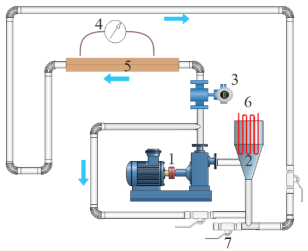1.Slurry pump; 2. Slurry tank; 3. Electromagnetic flow meter; 4. Differential pressure transducer; 5. Rectangular test component; 6.Coil heat exchanger; 7.Valve

(a) Schematic overview

## 1b.png(b) Lab circuit

Figure 1. Experiment setup

During the measurement, the loop containing rectangular test component was bypassed to control the flow rate. The volumetric flow rate of the slurry was recorded by an electromagnetic flow meter (full scale: 28m3/h; accuracy: 0.5%).

The pressure drops over the 1,400mm-long section was captured by differential pressure transmitters (full scale: 3,000Pa; accuracy: 0.2%). The measuring section is 900 mm away from the channel entrance and 212 mm away from the exit.

## 2.png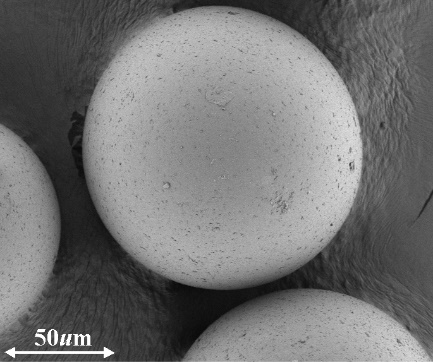Figure 2. Micrograph of the glass beads

The experiment adopts glass beads with density of 2,477 kg/m3. Figure 2 presents the micrograph of the glass beads acquired by field emission electronic microscope. It is observed that the glass beads are spherical in shape. The particle size distribution (Figure 3) was determined by laser scattering analyser. It can be seen that the mean diameter of the glass beads is 120.2um with a geometric standard variance of 1.27. The geometric standard variance is less than 1.5, indicating that the glass beads obey a narrow distribution .

## 3.png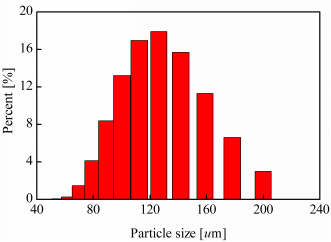Figure 3.  Size distribution of the particles

4. More Details About Paper Title and Author Information

Table 1. Physical and numerical parameters

 Parameter Values Slurry case A case B superficial flow velocity 1.0 m/s 1.0 m/s mean solid volume fraction 10% 20% Liquid phase (water) viscosity 8.0 × 10-4 Pas density 995.7 kgm-3 Solid phase (glass beads) case A case B number of particles 176 000 352 000 diameter 120.2 um density 2 477 kgm-3 sphericity 1.0 normal/tangential spring constant 1 000 Nm-1 normal/tangential restitution coefficient 0.6 coefficient of sliding friction 0.3 coefficient of rolling friction 0.005

During the simulation, periodic boundary conditions were applied in the length and width direction for all variables in both the CFD and DEM aspects. The pipe length was made as long as computationally tractable. Therefore, the computational domain is 100mm (X direction) in length, 1.0mm (Y direction) in width, and 12mm (Z direction) in height. The domain was divided into 800×8×96 structured elements along the X, Y, and Z directions, respectively. Since the element size is roughly equal to particle diameter, the divided particle volume method was adopted to obtain the interphase interaction force and the volume fraction field .

Two cases were analysed without changing any parameter but the mean solid volume fraction.

Monodisperse glass beads with diameter of 120.2um were selected for the simulation. The physical and numerical parameters are listed in Table 1.

5. Math

5.1 Model validation

## 4.png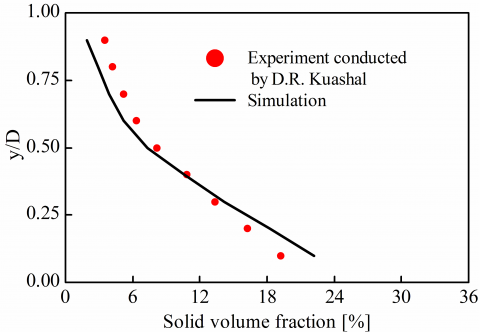Figure 4.  Comparison of the predicted and measured vertical solid distributions

Due to the narrowness of the channel, it is impossible to acquire the solid concentration along the vertical direction through the experiment (Section 3). Thus, the proposed model was validated by the experiment of D.R. Kuashal et al. The experiment is conducted in the tube with the length of 3m and the diameter of 54.9mm. During the model validation, both the pressure gradient and the vertical solid distribution were measured, and periodical boundary condition was applied along the length for both phases. The main parameters are set as follows: the surface slurry velocity was 1.0 m/s; the mean volume fraction of 125 um-diameter solids was 9.4%; the geometrical model was 274.5mm in length and 54.9mm in inner diameter; The liquid-solid interaction parameters are the same as those in Table 1.

The pressure drop was predicted to be 289 Pa/m, while the measured data was 261 Pa/m. According to the vertical solid distribution in Figure 4, the simulated data agree well with the experimental results. This means the proposed model accurately captured the key hydrodynamic properties of the slurry flows in horizontal channel.

5.2 Measurement units and numbers

Figure 5 illustrates the pressure drop per unit length against the flow velocity of the 12mm-tall horizontal rectangular channel. For the slurry flows, the pressure drop decreased monotonously with the reduction of the velocity until a critical value was reached. The critical value, called the critical deposition velocity, corresponds to the position of the bottom-most particles. The critical deposition velocity, positively correlated with the solid concentration, was 0.76m/s and 0.85m/s in cases A and B, respectively. Subsequently, the thickness of the static bed thickness gradually went up with further reduction of the flow velocity. When the bed thickness surpassed the cross-section height of the channel, the channel was clogged up with deposition. Therefore, the pressure drop is negatively correlated with the critical deposition velocity. The trend is different from that of a single-phase system. In addition, the pressure drop increased with solid volume fraction at any given slurry velocity, as more energy was consumed to keep more particles moving. The simulated results echo with the experimental data at different solid concentrations and flow velocities, indicating that the proposed model can make accurate predictions.

## 5.png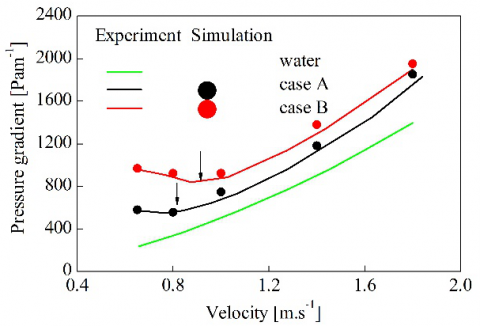Figure 5. Pressure drop per unit length versus the flow velocity

5.3 General flow behaviour

In the narrow horizontal channel, the particles moved vigorously with the fluid phase. Figure 6 shows the simulated solid flow regimes for two cases. Under the action of gravity, most particles migrated in the central and lower part of the channel. In the meantime, a dilute disperse phase and a dense cluster phase were formed. In the dispersed phase, the particles existed as independent entities. In the cluster phase, however, the particles clustered together and got enmeshed in the dispersed phase.

## 6.pngFigure 6. Simulated solid flow regimes: (a) solid volume fraction; (b) particle velocity; (c) coupling force imposed on the solid phase

It is hard to determine the shape of the dense clusters, for they changed continuously in the dynamic process. Some particles in dispersed phase were entrained into the cluster, and then accelerated by a greater coupling force to a speed faster than its neighbours. With the continued deformation of the cluster, some particles shifted from the cluster phase to dispersed phase. Moreover, there are visible irregular longitudinal waves resulted from the unsteady and chaotic movement of the solid phase.

5.4 Time-averaged behaviour

Whereas it is impossible to obtain relatively regular movement features from Figure 6, the time-averaged method was introduced to quantify the hydrodynamics. With the addition of the solid phase, a start-up procedure appeared until the pseudo-steady-state was reached. In the simulation, the pseudo-steady-state is observed at t = 1.2s. Thus, the data obtained in the first 1.2s were discarded, and the time-averaged analysis was performed on the data measured from 1.2s to 5s.

## 7.pngFigure 7. Time-averaged solid volume fraction along the vertical direction

Figure 7 displays the time-averaged solid concentration profiles along the vertical direction. As shown in the figure, the solid distributions are asymmetrical around the centreline of the channel. The degree of asymmetry decreased with the increase in solid concentration, owing to the interference enhancing effect among solid particles.

In addition, the maximum concentration points situated above the bottom of the channel. This distribution is attributable to the existence of a hydrodynamic lift force, which repelled particles from the walls. Similar phenomenon was observed in other experiments [22, 23]. The effect of the hydrodynamic lift force largely depends on the near-wall liquid-solid interaction. There are two mechanisms of the interaction: the viscous “liquid-like” interaction and mechanical interaction . For fine-particle slurries, the particles can be enclosed within the viscous sublayer, pushing up the density and the viscosity of the carrying fluid. For coarse-particle slurries, the particles are too large to enter the viscous sub-layer or impinge with channel walls. Therefore, the solid distribution will exhibit an anomalous pattern if the ratio of particle diameter to the size of viscous sub-layer is excessively large. To quantify this behaviour, a dimensionless particle size was proposed for general slurry flows :

$d_{p}^{+}=\frac{\rho_{f} d_{p} u_{f}^{*}}{\mu_{f}}$     (9)

where $u_{f}^{*}$ is the friction velocity of the fluid, i.e. the square root of the ratio between fluid wall shear stress and fluid density. According to the above equation, the time-averaged value of $d_{p}^{+}$ is calculated as 10.24 for case A and 10.03 for case B. Both values are greater than 9.0, implying that near-wall particles were not trapped in the thin viscous sublayer, but interacted with the viscous-turbulent interface . Then, the interaction drove the particles away from the bottom wall, resulting in a particle-lean region.

Figure 8 shows the time-averaged solid velocity distribution along the vertical direction. The velocity profiles are asymmetrical, with maximum values falling in the upper part of the centreline. The distribution pattern is explained as follows. As more particles travelled in the lower region, more energy was dissipated, leading to lower velocity. With the increase in solid concentration, the number of particles grew faster in the lower part than in the upper part. Thus, the velocity profile in the upper part further increased, while that in the lower part declined accordingly.

## 8.pngFigure 8. Time-averaged solid velocity along the vertical direction

5.5 Statistics properties

Statistics were extracted to gain more insights on slurry flow features. The standard variances of solid concentration and solid velocity are respectively presented in Figure 9 and Figure 10, where u, v and w are the velocity components along the X, Y and Z directions, respectively.

For simplicity, three distinct layers, denoted as Regions I, II and III, were partitioned. Region I lies near the bottom wall. The dominant force in this region is the hydrodynamic lift force (Section 5.4). The largest standard variance of solid concentration and u belonged to this region. Located right above Region I, Region II witnessed the most violent fluctuation of v because it contains highly collisional particles and stores high liquid turbulence energy. Due to the successive passing of the dense phase and the dilute phase, there is little change to the standard variance of solid concentration and u across Region II. Region III is situated on top of both Regions I and II. In this region, the solid concentration reached the minimum value. The standard variance dropped rapidly to zero because the slurry flows are dilute in nature.

The particle velocity has a direct bearing on solid distribution. The profiles of the covariance between solid velocity and solid concentration along the vertical direction are presented in Figure 11. The $\left\langle\boldsymbol{u}_{p}^{\prime} \alpha_{p}^{\prime}\right\rangle$ was small in Region III under the presence of few solid particles, while $\left\langle v_{p}^{\prime} \alpha_{p}^{\prime}\right\rangle$ was uncorrelated over the entire length of vertical direction. In Regions I and II, the $\left\langle w_{p}^{\prime} \alpha_{p}^{\prime}\right\rangle$ remained positive, a signal of the elevation of dense clusters. Meanwhile, the particles in these clusters were accelerated horizontally to a speed faster than that of the discrete particles, leading to a positive $\left\langle u_{p}^{\prime} \alpha_{p}^{\prime}\right\rangle$. These behaviours can be explained by the Kelvin-Helmholtz instabilities under high shear in slurry velocity and steep gradient in solid concentration.

## 9.pngFigure 9.  Standard variance profiles of solid concentration

## 10.png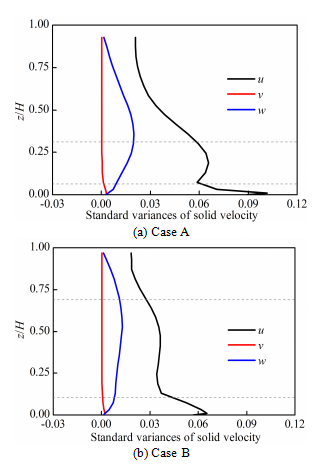Figure 10. Standard variance profiles of solid velocity

## 11.png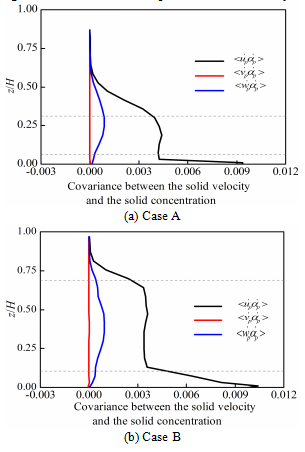Figure 11. Covariance between solid velocity and solid concentration

5.6 Solid dispersion

Solid dispersion is closely related to particle transfer characteristic, especially in the procedure of heat transfer . The solid dispersion intensity can be described with the solid dispersion coefficient. In general, the solid dispersion coefficient is evaluated either by the micro method or the macro method . The former is grounded on particle displacement, and the latter on the solid concentration and a Fichian-type diffusion equation. The micro method was selected for this research. For a specific particle i, the solid dispersion coefficient can be evaluated with Einstein’s equations:

$D_{i}=\frac{\left(\Delta r_{i}\right)^{2}}{2 \Delta t}$     (10)

where Di and ∆ri are the local dispersion coefficient and displacement of particle i along a specific direction, respectively; ∆t is the time interval. To disclose the local solid dispersion property, the dispersion coefficient can be calculated by averaging the dispersion coefficients of all particles in the region:

$\overline{D}=\frac{1}{N_{p}} \sum_{i=1}^{N_{p}} \frac{\left(\Delta r_{i}\right)^{2}}{2 \Delta t}$     (11)

Figure 12 shows the time-varying profiles of the vertical solid dispersion coefficient. Overall, the dispersion coefficient fluctuated around a constant value because of the chaotic and turbulent motion. Comparatively speaking, the mean dispersion coefficient of Case B is slightly smaller than that of Case A. A possible explanation lies in the suppression of solid vertical motion by the increase in solid concentration. Similarly, the particles in Region III experienced more intense vertical dispersion than those in Region I in either case 1 or case 2.

## 12.png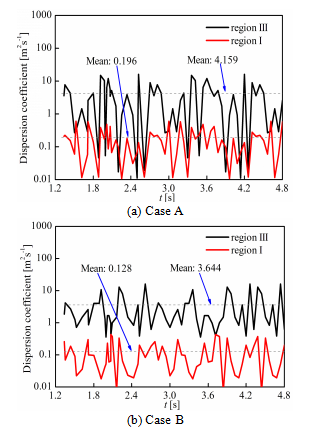Figure 12. Time-varying profiles of vertical solid dispersion coefficient

6. Conclusions

Through experiment and numerical simulation, this paper investigates the hydrodynamics of slurry flows in a 12-mm tall horizontal rectangular channel. Based on the acquired slurry flow features, some conclusions were derived as follows:

(1) A dilute disperse phase and a dense cluster phase were formed. The dense cluster phase was elevated and moved faster than the surrounding dispersed phase.

(2) In the vertical direction, the solid concentration is positively correlated with the degree of asymmetry of solid velocity profiles, but negatively with that of solid concentration profiles. The vertical solid dispersion became less intense with the increase of solid concentration.

(3) Three distinct regions were identified in the slurry flows: the near-wall region, the highly-collisional shear flow region, and the dilute particle-laden region. The standard variances of streamwise solid velocity and solid concentration peaked in the near-wall region, as particles were away from the bottom wall by hydrodynamic lift force; the variances remained stable in the highly-collisional shear flow region, and plunged rapidly to zero in the dilute particle-laden region.

References

 Kuashal D.R., Tomita Y. (2007). Experimental investigation for near-wall lift of coarser particles in slurry pipeline using γ-ray densitometer, Powder Technology, Vol. 172, No. 3, pp. 177-187. DOI: 10.1016/j.powtec.2006.11.020

 Li G.N., Sun S.K., Liu H.T., Zheng T.G., Zhang C. (2017). Water profiles in vertical slot fishways without central baffle, International Journal of Heat and Technology, Vol. 35, No. 1, pp. 191-195. DOI: 10.18280/ijht.350125

 Gillies R.G., Shook C.A., Xu J. (2010). Modelling heterogeneous slurry flows at high velocities, Canadian Journal of Chemical Engineering, Vol. 82, No. 5, pp. 1060-1065. DOI: 10.1002/cjce.5450820523

 Ravelet F., Bakir F., Khelladi S., Rey R. (2013). Experimental study of hydraulic transport of large particles in horizontal pipes, Experimental Thermal & Fluid Science, Vol. 45, No. 2, pp. 187-197. DOI: 10.1016/j.expthermflusci.2012.11.003

 Roco M.C., Shook C.A. (2010). Modeling of Slurry Flow: The effect of particle size, Canadian Journal of Chemical Engineering, Vol. 61, No. 4, pp. 494-503. DOI: 10.1002/cjce.5450610402

 Dayal P.A.S., Raju G.S.N., Mishra S. (2016). Pattern synthesis using accelerated particle swarm optimization, Modelling, Measurement and Control A, Vol. 89, No. 1, pp. 58-76.

 Capecelatro J., Desjardins O. (2013). Eulerian-Lagrangian modeling of turbulent liquid-solid slurries in horizontal pipes, International Journal of Multiphase Flow, Vol. 55, pp. 64-79. DOI: 10.1016/j.ijmultiphaseflow.2013.04.006

 Hashemi S.A., Sadighian A., Shah S.I.A., Sanders R.S. (2014). Solid velocity and concentration fluctuations in highly concentrated liquid-solid (slurry) pipe flows, International Journal of Multiphase Flow, Vol. 66, pp. 46-61. DOI: 10.1016/j.ijmultiphaseflow.2014.06.007

 Messa G.V., Malavasi S. (2015). Improvements in the numerical prediction of fully-suspended slurry flow in horizontal pipes, Powder Technology, Vol. 270, No. Part A, pp. 358-367. DOI: 10.1016/j.powtec.2014.10.027

 Li Z.X., Li C., Jue Z. (2016). Multi-objective particles warm optimization algorithm for recommender system, Advances in Modelling and Analysis B, Vol. 59, No. 1, pp. 189-200.

 Messa G.V., Malin M., Malavasi S. (2014). Numerical prediction of fully-suspended slurry flow in horizontal pipes, Powder Technology, Vol. 256, pp. 61-70. DOI: 10.1016/j.powtec.2014.02.005

 Thomas A.D. (1979). Predicting the deposit velocity for horizontal turbulent pipe flow of slurries, International Journal of Multiphase Flow, Vol. 5, No. 2, pp. 113-129. DOI: 10.1016/0301-9322(79)90040-5

 Gillies R.G., Schaan J., Sumner R.J., Mckibben M.J., Shook C.A. (2010). Deposition velocities for newtonian slurries in turbulent flow, Canadian Journal of Chemical Engineering, Vol. 78, No. 4, pp. 704-708. DOI: 10.1002/cjce.5450780412

 Matousek V. (2009). Predictive model for frictional pressure drop in settling-slurry pipe with stationary deposit, Powder Technology, Vol. 192, No. 3, pp. 367-374. DOI: 10.1016/j.powtec.2009.01.017

 Kumar B., Singh S.N. (2015). Analytical studies on the hydraulic performance of chevron type plate heat exchanger, International Journal of Heat and Technology, Vol. 33, No. 1, pp. 17-24. DOI: 10.18280/ijht.330103

 Zhang X., Li H.W., Feng X.H. (2017). Rheological Properties of steam-exploded corn straw slurry and enzyme hydrolysate, Chemical Engineering Transactions, Vol. 59, pp. 277-282. DOI: 10.3303/CET1759047

 Zhang J., Tang T., Shi F. (2016). Study on influence of ball seat on pressure drop in horizontal open hole staged fracturing, Chemical Engineering Transactions, Vol. 59, pp. 145-150. DOI: 10.3303/CET1651025

 Du Y., Chen Q.X., Mao N. (2015). The research of variable production line into multi-channel, Academic Journal of Manufacturing Engineering, Vol.13, No.4, pp. 6-12.

 Feng Y.Q., Yu A.B. (2004). Assessment of model formulations in the discrete particle simulation of gas-solid flow, Industrial & Engineering Chemistry Research, Vol. 43, No. 26, pp. 8378-8390. DOI: 10.1021/ie049387v

 Kuashal D.R., Sato K., Toyota T., Funatsu K., Tomita Y. (2005). Effect of particle size distribution on pressure drop and concentration profile in pipeline flow of highly concentrated slurry, International Journal of Multiphase Flow, Vol. 31, No. 7, pp. 809-823. DOI: 10.1016/j.ijmultiphaseflow.2005.03.003

 Sun R., Xiao H. (2015). Diffusion-based coarse graining in hybrid continuum–discrete solvers: Applications in CFD–DEM, International Journal of Multiphase Flow, Vol. 72, No. 23, pp. 233-247. DOI: 10.1016/j.ijmultiphaseflow.2015.08.014

 Shook C.A., Daniel S.M., Scott J.A., Holgate J.P. (1968). Flow of suspensions in pipelines (Part 2: Two mechanisms of particle suspension), Canadian Journal of Chemical Engineering, Vol. 46, No. 4, pp. 238-244. DOI: 10.1002/cjce.5450460405

 Pendyala R., Wong Y.S., Ilyas S.U. (2015). CFD simulations of natural convection heat transfer in enclosures with varying aspect ratios, Chemical Engineering Transactions, Vol. 45, pp. 793-798. DOI: 10.3303/CET1545133

 Matousek V. (2005). Research developments in pipeline transport of settling slurries, Powder technology, Vol. 156, No. 1, pp. 43-51. DOI: 10.1016/j.powtec.2005.05.054

 Wilson K.C., Sanders R.S., Gillies R.G., Shook C.A. (2010). Verification of the near-wall model for slurry flow, Powder Technology, Vol. 197, No. 3, pp. 247-253. DOI: 10.1016/j.powtec.2009.09.023

 Alam M.S., Islam T., Rahman M.M. (2015). Unsteady hydromagnetic forced convective heat transfer flow of a micropolar fluid along a porous wedge with convective surface boundary condition, International Journal of Heat and Technology, Vol. 33, No. 2, pp. 115-122. DOI: 10.18280/ijht.330219

 Liu D., Chen X. (2010). Lateral solids dispersion coefficient in large-scale fluidized beds, Combustion & Flame, Vol. 157, No. 11, pp. 2116-2124. DOI: 10.1016/j.combustflame.2010.04.020